# vs.eyeandcontacts.com

## Chapter 2 Linear Equations in One Variable Exercise 2.4

Question 1: Amina thinks of a number and subtracts 5/2 from it. She multiplies the result by 8. The result now obtained is 3 times the same number she thought of. What is the number?

Let the number be x.
Given (x - 5/2) × 8 = 3x
= x - 5/2 = 3/8x
= x/1 - 3x/8 = 5/2
= 8x - 3x/8 = 5/2
= 5x/8 = 5/2
= x = 8/2
= x = 4
Verification
= (4 - 5/2) × 8 = 3 × 4
= (8 - 5/2) × 8 = 12
= 3/2 × 8 = 12
= 3 × 4 = 12
= 12 = 12
Therefore, the number is 12.

Question 2: A positive number is 5 times another number. If 21 is added to both the numbers, then one of the new numbers becomes twice the other new number. What are the numbers?

Let another number be x.
Given the number = 5x
Numbers are
x + 21
5x + 21= x = 7
= 5x = 7 × 5 = 35
= x + 21 = 28
= 5x + 21 = 5 × 7 + 21 = 56
Therefore, the numbers are 28 and 56.

Question 3: Sum of the digits of a two-digit number is 9. When we interchange the digits, it is found that the resulting new number is greater than the original number by 27. What is the two-digit number?

Let the units digit be x and tens digit be 9 - x.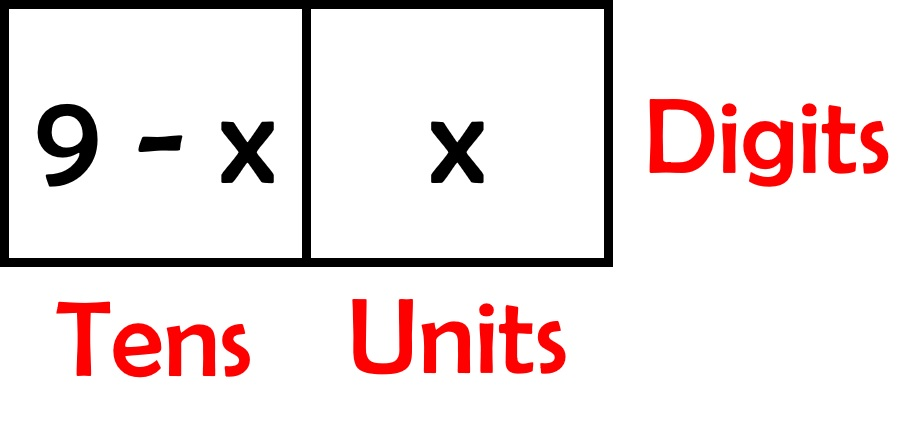Number
= 10(9 - x) + 1(x)
= 90 - 10x + x
= 90 - 9xNumber
= 10(x) + 1(9 - x)
= 10x + 9 - x
= 9x + 9
Given
= 9x - 9 - (90 - 9x) = 27
= 9x + 9 - 90 + 9x = 27
= 18x - 81 = 27
= 18x = 27 + 81
= 18x = 108
= x = 108/18
= x = 6
Numbers areTherefore, the number is 36, if it is interchanged it becomes 63.

Question 4: One of the two digits of a two-digit number is three times the other digit. If you interchange the digits of this two-digit number and add the resulting number to the original number, you get 88. What is the original number?
Let the units digit be x and tens digit be 3x.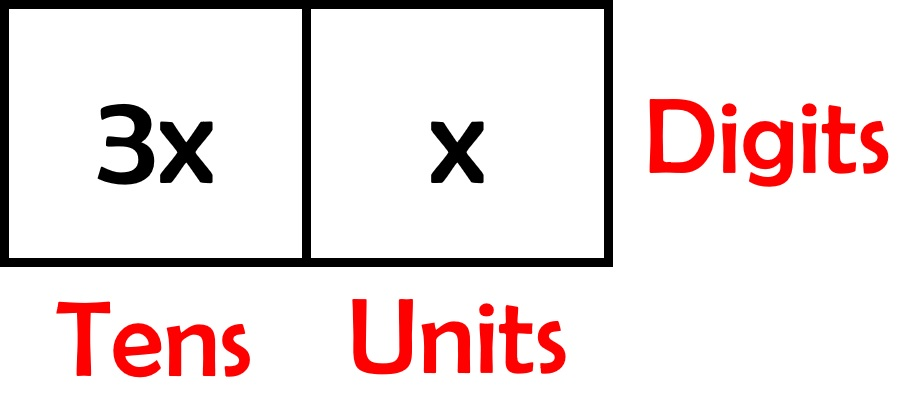Number
= 10(3x) + 1(x)
= 30x + x
= 31x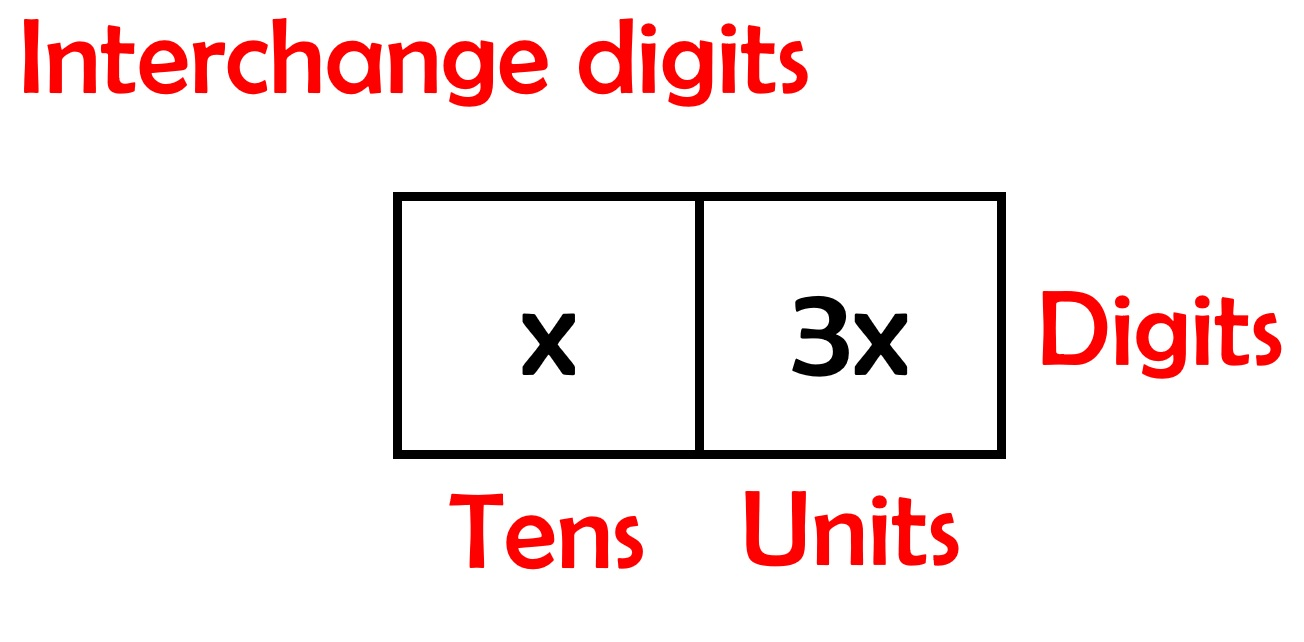Number
= 10(x) + 1(3x)
= 10x + 3x
= 13x
= 31x + 13x = 88
= 44x = 88
= x = 88/44
= x = 2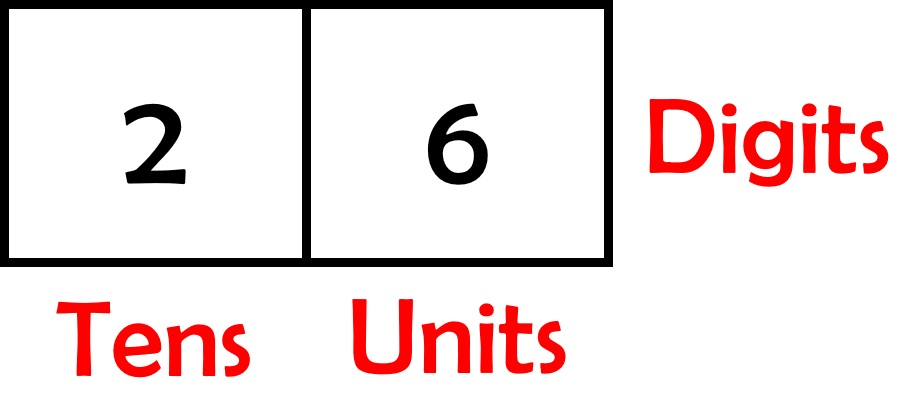Therefore, the number is 26, if it is interchanged it becomes 62.

Question 5: Shobo’s mother’s present age is six times Shobo’s present age. Shobo’s age five years from now will be one third of his mother’s present age. What are their present ages?= x + 5 = 1/3 (6x)
= x + 5 = 2x
= 5 = 2x - x
= x = 5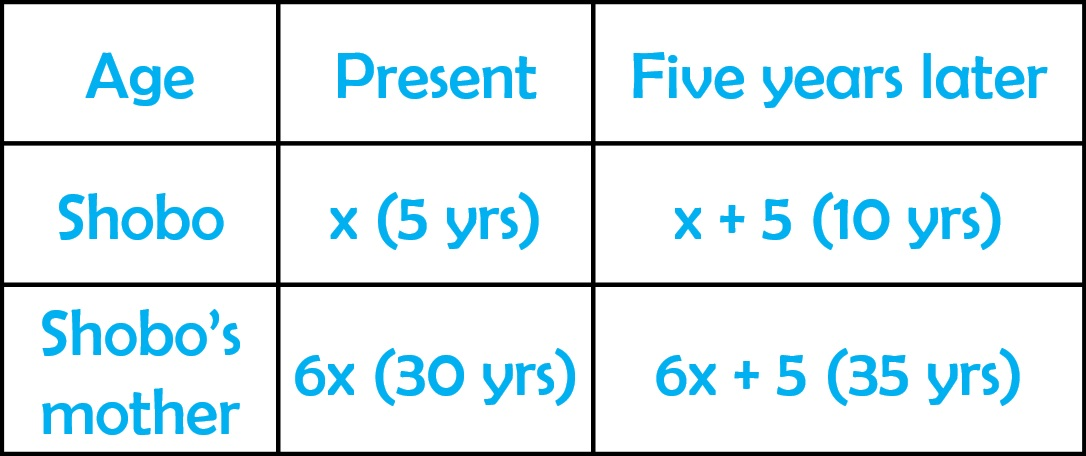Therefore, Shobo’s present age is 5 years.

Question 6: There is a narrow rectangular plot, reserved for a school, in Mahuli village. The length and breadth of the plot are in the ratio 11:4. At the rate ₹100 per metre it will cost the village panchayat ₹75000 to fence the plot. What are the dimensions of the plot?Let the length of narrow rectangular plot be 11x. Let the breadth of narrow rectangular plot be 4x.
Perimeter of rectangle = 2 × (l + b)
= 2(l + b) × 100 = 75000
= 2(11x + 4x) × 100 = 75000
= 2(15x) × 100 = 75000
= 3000x = 75000
= x = 75000/3000
= x = 25
Length = 11x = 25 × 11 = 275 m
Breadth = 4x = 25 × 4 = 100 m
Therefore, the length of the plot is 275 m while of the breadth of plot is 100 m.

Question 7: Hasan buys two kinds of cloth materials for school uniforms, shirt material that costs him ₹50 per metre and trouser material that costs him ₹90 per metre. For every 3 meters of the shirt material he buys 2 metres of the trouser material. He sells the materials at 12% and 10% profit respectively. His total sale is ₹36,600. How much trouser material did he buy?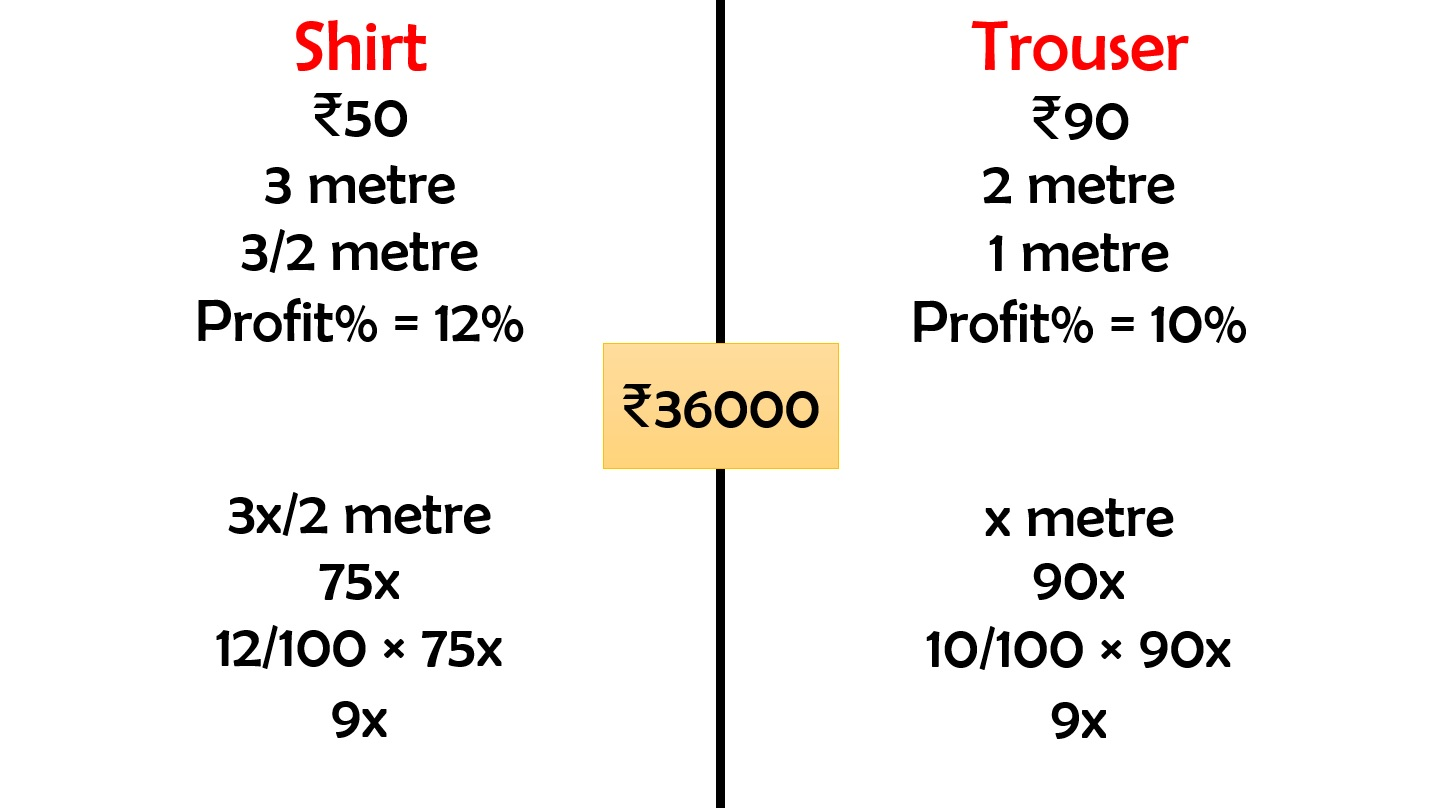Shirt
= 75x + 9x
= 84x
Trouser
= 90x + 9x
= 99x
= 84x + 99x = 36000
= 183x = 36000
= x = 36000/183
= x = 200
Trouser material
= x = 200 m
Shirt material
= 3x/2 = 3 × 200/2 = 600/2 = 300 m
Therefore, he brought 200 metres of trouser material, brought 300 m of shirt material.

Question 8: Half of a herd of deer are grazing in the field and three fourths of the remaining are playing nearby. The rest 9 are drinking water from the pond. Find the number of deer in the herd.

Number of deer in herd = x
Grazing = x/2
Remaining = x - x/2 = x/2
Playing = 3/4 (x/2) = 3x/8
Drinking water = 9
= x/2 + 3x/8 + 9/1 = x
= 4x + 3x + 72/8 = x
= 7x + 72 = 8x
= 72 = 8x - 7x
= x = 72
So, number of deer
in the herd = x = 72
grazing = x/2 = 72/2 = 36
remaining = x/2 = 72/2 = 36
playing = 3x/8 = 216/8 = 27
drinking water = 9 = 9
Therefore, the total number of deer in the herd is 72.

Question 9: A grandfather is ten times older than his granddaughter. He is also 54 years older than her. Find their present ages.

Let granddaughter’s age be x years.= 10x - x = 54
= 9x = 54
= x = 54/9
= x = 6Therefore, grandfather’s age is 60 years, while the granddaughter’s age is 6 years.

Question 10: Aman’s age is three times his son’s age. Ten years ago he was five times his son’s age. Find their present ages.

Let son’s age be x years.= 3x - 10 = 5(x - 10)
= 3x - 10 = 5x - 50
= -10 + 50 = 5x - 3x
= 40 = 2x
= x = 40/2
= x = 20Therefore, Aman’s age is 60 years. His son’s age is 20 years.